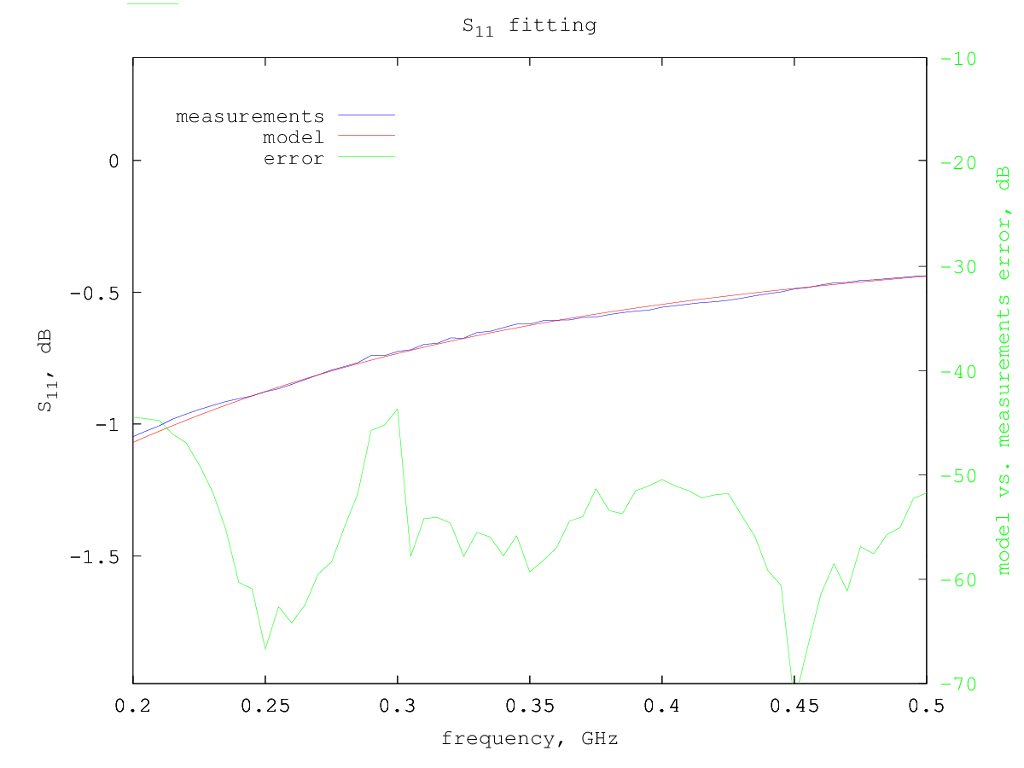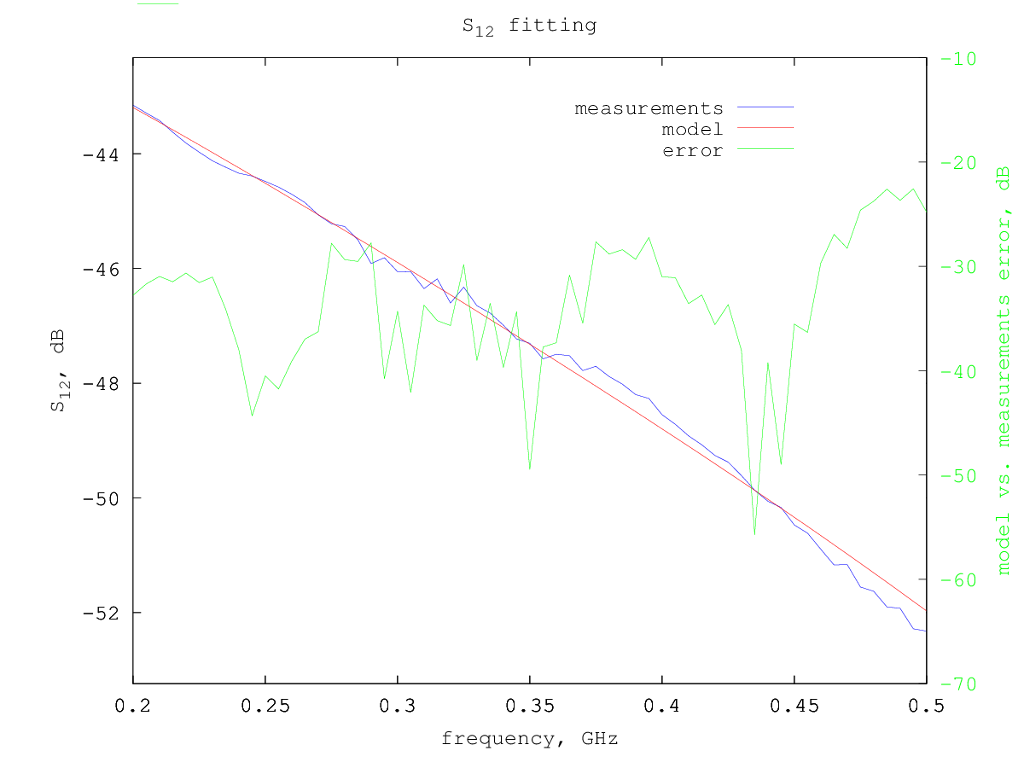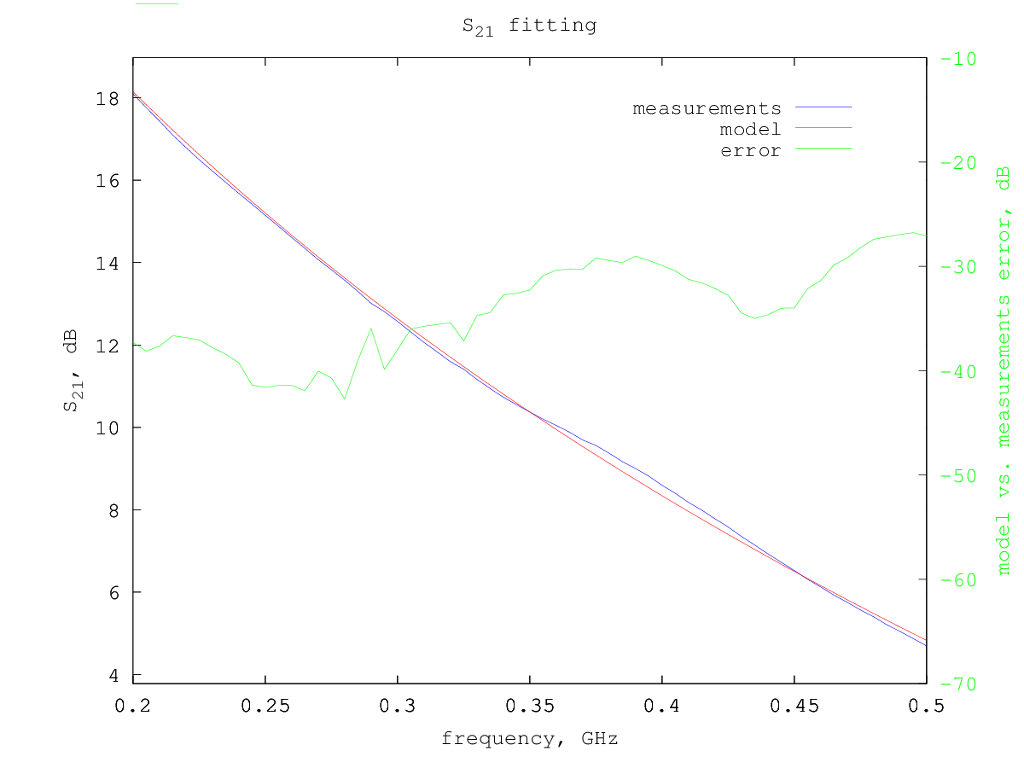# Freescale AFT05MS031N LDMOS model

Under construction...

The Freescale AFT05MS031N is a low power LDMOS.

# Model for Vdd=13.6 V and Idd= 500 mA

Typical S-parameters for this device can be found on the NXP/Freescale web site, for a supply voltage of 13.6 V and a drain current of 500 mA.

Using the extraction method previously described, considering only the S-parameters from 200 MHz to 500 MHz, the following small-signal model parameters are determined:

```Rg = 0.58 ohm
Rd = 0.22 ohm
Rs = 0.01 ohm
Lg = 0.4 nH
Ld = 0.24 nH
Ls = 0.014 nH
Cgs = 98.3 pF
Cds = 46.2 pF
Cgd = 1.6 pF
gm = 2.26 S
gds = 1.6 mS
```
So the equivalent small-signal circuit for this LDMOS at 500 mA drain current is the following:Smith chart with measured vs. model S-parameters:S-parameters fitting details - fitted region is in white background: